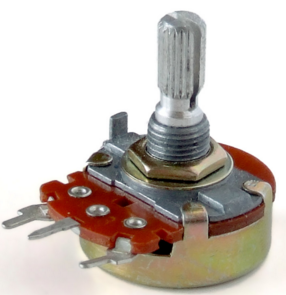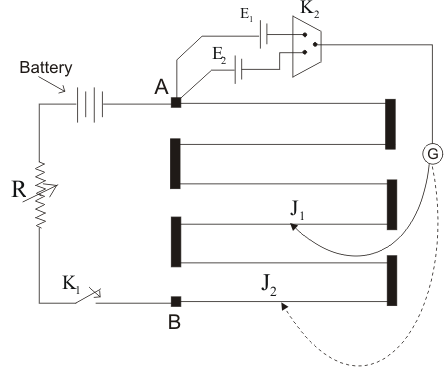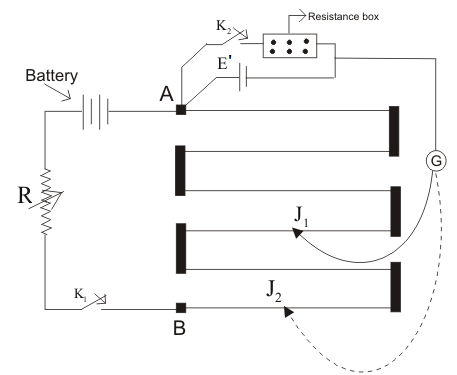# Potentiometer

It is an electrical device that is used to measure the emf of a cell in very precise manner. It can also compare the emf of two cells and find the internal resistance of two cells.Potentiometer

Principle:

When constant amount of current is passed through the wire of uniform cross sectional area, the potential difference across the wire is directly proportional to the length of the wire.

We know,

V = IR

or, V = I . ρl/A

Here, I,  ρ and A are constant.

∴ V ∝ l

Uses of potentiometer:Circuit diagram of comparison of emf of two cells

1. Comparison of emfs of two cells and determination of emf of two cells:

To compare the emf of two cells, a driver cell is connected across the potentiometer wire along the rheostat and a switch as shown in figure. The cell whose emf are to be compared are connected to the wire and to the two way key as shown in figure.

A galvanometer is connected between the key and a jockey as shown in figure. Now, on closing the key (K1), remaining (K2) opens. Now, on moving the jockey along the wire, suppose at point  C, the galvanometer shows no deflection. Now,

E1 = VAC

But, VAC ∝ lAC

or, E1 ∝ lAC

E1 = K.lAC……(i)

Now, when the key K1 is opened and key K2  is closed, then the jockey is moved along the wire. Suppose at point C, the galvanometer shows null deflection.

E2 = VAC’

But, VAC’ ∝ lAC’

or, E1′ ∝ lAC’

E1′ = K.lAC’……(ii)

Now, dividing equation (i) by (ii),

$$\frac{\text{E}_1}{\text{E}_2} = \frac{\text{l}_{AC}}{\text{l}_{AC’}}$$

From the above relation, we can compare the emf of two cells.

2. Potentiometer is also used to find the internal resistance of a cell.Circuit diagram for determination of emf of a cell

A driver cell having e.m.f. E’ is connected across a wire of potentiometer along with the rheostat and a switch as shown in figure. The cell whose internal resistance is to be determined is connected between the positive end of the wire. A resistance is connected across the cell along with a key (K) as shown in figure. Now, the galvanometer is connected to the jockey.

Now, on closing the switch, the key (K) opens. The jockey is moved along the wire. Suppose at point C, the galvanometer shows null deflection.

Therefore,

E = VAC

We know,

But, VAC ∝ lAC

or, E ∝ lAC

or, E = k. lAC……(i)

Now, the key is closed and jockey is moved along the wire. Suppose, at point ‘C’ the galvanometer shows null deflection.

So, terminal p.d. of the cell (V) = VAC’

We know,

But, VAC’ ∝ lAC’

V ∝ lAC’

V = K.lAC’……(ii)

Now, dividing equation (i) by (ii), we get:

$$\frac{\text{E}}{\text{V}} = \frac{\text{l}_{AC}}{\text{l}_{AC’}}$$

$$\text{or,} \frac{\text{IR + Ir}}{\text{IR}} = \frac{\text{l}_{AC}}{\text{l}_{AC’}}$$

$$\text{or,} 1 + \frac{\text{r}}{\text{R}} = \frac{\text{l}_{AC}}{\text{l}_{AC’}}$$

$$\text{or,}1 + \frac{\text{r}}{\text{R}} = \frac{\text{l}_1}{\text{l}_2}$$

$$\text{or,} \frac{\text{r}}{\text{R}} = \frac{\text{l}_1}{\text{l}_2} -1$$

$$\text{or, r} = R \left(\frac{\text{l}_1}{\text{l}_2} -1 \right)$$

$$\text{or, r} = R \left(\frac{\text{l}_2 – \text{l}_1}{\text{l}_2} \right)$$

From the equation above, the internal resistance of the cell can be determined.

Do you like this article ? If yes then like otherwise dislike :